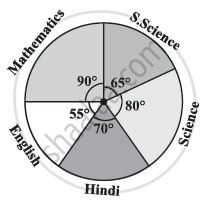Share

# The Adjoining Pie Chart Gives the Marks Scored in an Examination by a Student in Hindi, English, Mathematics, Social Science and Science. If the Total Marks Obtained by the Students Were 540, Answer the Following Questions - CBSE Class 8 - Mathematics

ConceptCircle Graph Or Pie Chart - Drawing Pie Charts

#### Question

The adjoining pie chart gives the marks scored in an examination by a student in Hindi, English, Mathematics, Social Science and Science. If the total marks obtained by the students were 540, answer the following questions.

1) In which subject did the student score 105 marks?

(Hint: For 540 marks, the central angle = 360°. So, for 105 marks, what is the central angle?)

2) How many more marks were obtained by the student in Mathematics than in Hindi?

3) Examine whether the sum of the marks obtained in Social Science and Mathematics is more than that in Science and Hindi.

(Hint: Just study the central angles)#### Solution

1) Total marks obtained by the student are 540. Hence, 540 marks represent 360º. The central angle for 105 marks has to be calculated.

Central angle for 105 marks = 105/540 xx 360^@ = 70^@

Hindi is the subject having its central angle as 70º.

Therefore, the student scored 105 marks in Hindi.

2) Difference between the central angles of Mathematics and Hindi

= 90º − 70º = 20º

Marks for 20º central angle =20^@/360^@ xx 540 = 30

There is a difference of 30 marks between the score obtained in Mathematics and Hindi.

Therefore, 30 more marks were obtained by the student in Mathematics than in Hindi.

3) Sum of central angles of Social Science and Mathematics

= 90° + 65° = 155°

Sum of central angles of Science and Hindi = 80° + 70° = 150°

The sum of the central angles for Social Science and Mathematics is more than that of Science and Hindi. Therefore, the student scored more in Social Science and Mathematics than in Science and Hindi

Is there an error in this question or solution?

#### APPEARS IN

NCERT Solution for Mathematics Textbook for Class 8 (2018 to Current)
Chapter 5: Data Handling
Ex. 5.20 | Q: 4 | Page no. 82

#### Video TutorialsVIEW ALL 

Solution The Adjoining Pie Chart Gives the Marks Scored in an Examination by a Student in Hindi, English, Mathematics, Social Science and Science. If the Total Marks Obtained by the Students Were 540, Answer the Following Questions Concept: Circle Graph Or Pie Chart - Drawing Pie Charts.
S